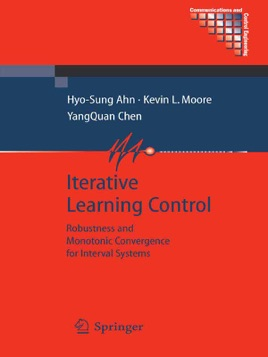• \$149.99

## Publisher Description

This monograph studies the design of robust, monotonically-convergent iterative learning controllers for discrete-time systems. Two key problems with the fundamentals of iterative learning control (ILC) design as treated by existing work are: first, many ILC design strategies assume nominal knowledge of the system to be controlled and; second, it is well-known that many ILC algorithms do not produce monotonic convergence, though in applications monotonic convergence is often essential.

Iterative Learning Control takes account of the recently-developed comprehensive approach to robust ILC analysis and design established to handle the situation where the plant model is uncertain. Considering ILC in the iteration domain, it presents a unified analysis and design framework that enables designers to consider both robustness and monotonic convergence for typical uncertainty models, including parametric interval uncertainties, iteration-domain frequency uncertainty, and iteration-domain stochastic uncertainty. Topics include:

• Use of a lifting technique to convert the two-dimensional ILC system, which has dynamics in both the time and iteration domains, into the supervector framework, which yields a one-dimensional system, with dynamics only in the iteration domain.

• Development of iteration-domain uncertainty models in the supervector framework.

• ILC design for monotonic convergence when the plant is subject to parametric interval uncertainty in its Markov matrix.

• An algebraic H-infinity design methodology for ILC design when the plant is subject to iteration-domain frequency uncertainty.

• Development of Kalman-filter-based ILC algorithms when the plant is subject to iteration-domain stochastic uncertainties.

• Analytical determination of the base-line error of ILC algorithms.

• Solutions to three fundamental robust interval computational problems (used as basic tools for designing robust ILC controllers): finding the maximum singular value of an interval matrix, determining the robust stability of interval polynomial matrix, and obtaining the power of an interval matrix.

Iterative Learning Control will be of great interest to academic researchers in control theory and to industrial control engineers working in robotics-oriented manufacturing and batch-processing-based industries. Graduate students of intelligent control will also find this volume instructive.